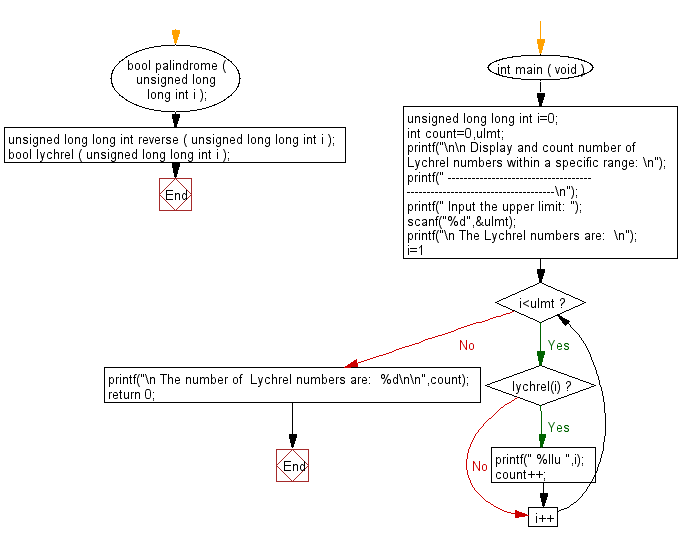﻿ C Program: Count the Lychrel numbers within a range# C Exercises: Display and count the number of Lychrel numbers within a specific range.

## C Numbers: Exercise-9 with Solution

Write a program in C to display and count the number of Lychrel numbers within a specific range(from 1 to a specific upper limit).

Test Data
Input the upper limit: 1000

Sample Solution:

C Code:

``````# include <stdio.h>
# include <stdbool.h>
# include <stdlib.h>

bool palindrome ( unsigned long long int i );
unsigned long long int reverse ( unsigned long long int i );
bool lychrel ( unsigned long long int i );

int main ( void )
{
unsigned long long int i=0;
int count=0,ulmt;
printf("\n\n Display and count number of Lychrel numbers within a specific range: \n");
printf(" -------------------------------------------------------------------------\n");
printf(" Input the upper limit: ");
scanf("%d",&ulmt);
printf("\n The Lychrel numbers are:  \n");
for(i=1;i<ulmt;i++)
{
if(lychrel(i))
{
printf(" %llu ",i);
count++;
}
}
printf("\n The number of  Lychrel numbers are:  %d\n\n",count);
return 0;
}
bool lychrel ( unsigned long long int i )
{
int j; /*iteration counter*/
bool lychrel = true;
i = i + reverse ( i );

for ( j = 1; j <= 30 ; j++ )
{
if ( palindrome ( i ) )
{
lychrel = false;
break;
}
i = i + reverse ( i );
}

return lychrel;
}
unsigned long long int reverse ( unsigned long long int i )
{
unsigned long long int ret = 0;
while ( i != 0 )
{
ret *= 10;
ret += i % 10;
i /= 10;
}
return ret;
}

bool palindrome ( unsigned long long int i )
{
return ( i == reverse ( i ) );
}
```
```

Sample Output:

``` Input the upper limit: 1000

The Lychrel numbers are:
196  295  394  493  592  689  691  788  790  879  887  978  986
The number of  Lychrel numbers are:  13
```

Pictorial Presentation:Flowchart:C Programming Code Editor:

What is the difficulty level of this exercise?

Test your Programming skills with w3resource's quiz.

﻿

## C Programming: Tips of the Day

Why do C and C++ compilers allow array lengths in function signatures when they're never enforced?

It is a quirk of the syntax for passing arrays to functions.

Actually it is not possible to pass an array in C. If you write syntax that looks like it should pass the array, what actually happens is that a pointer to the first element of the array is passed instead.

Since the pointer does not include any length information, the contents of your [] in the function formal parameter list are actually ignored.

Ref : https://bit.ly/3fhlvdH